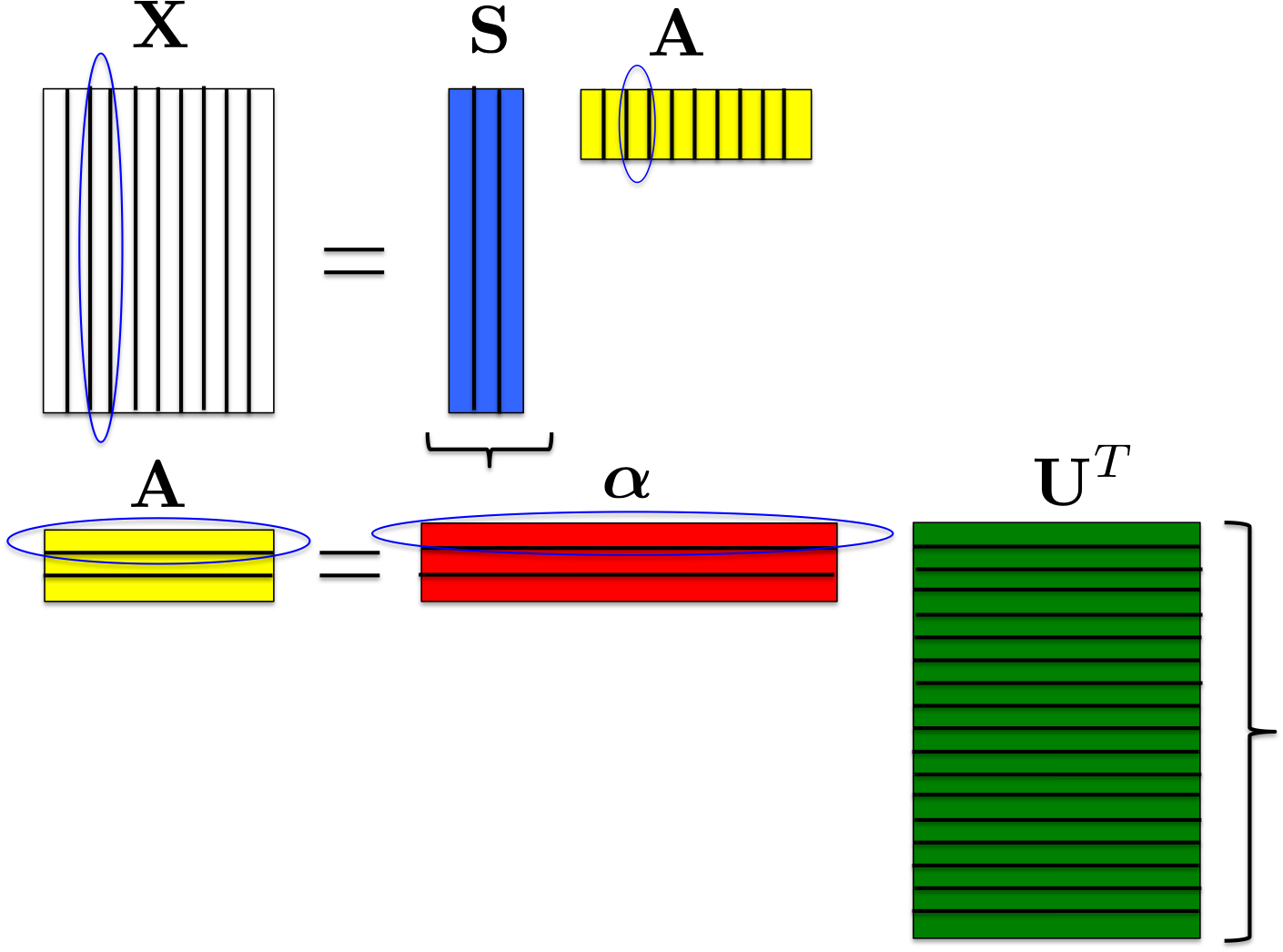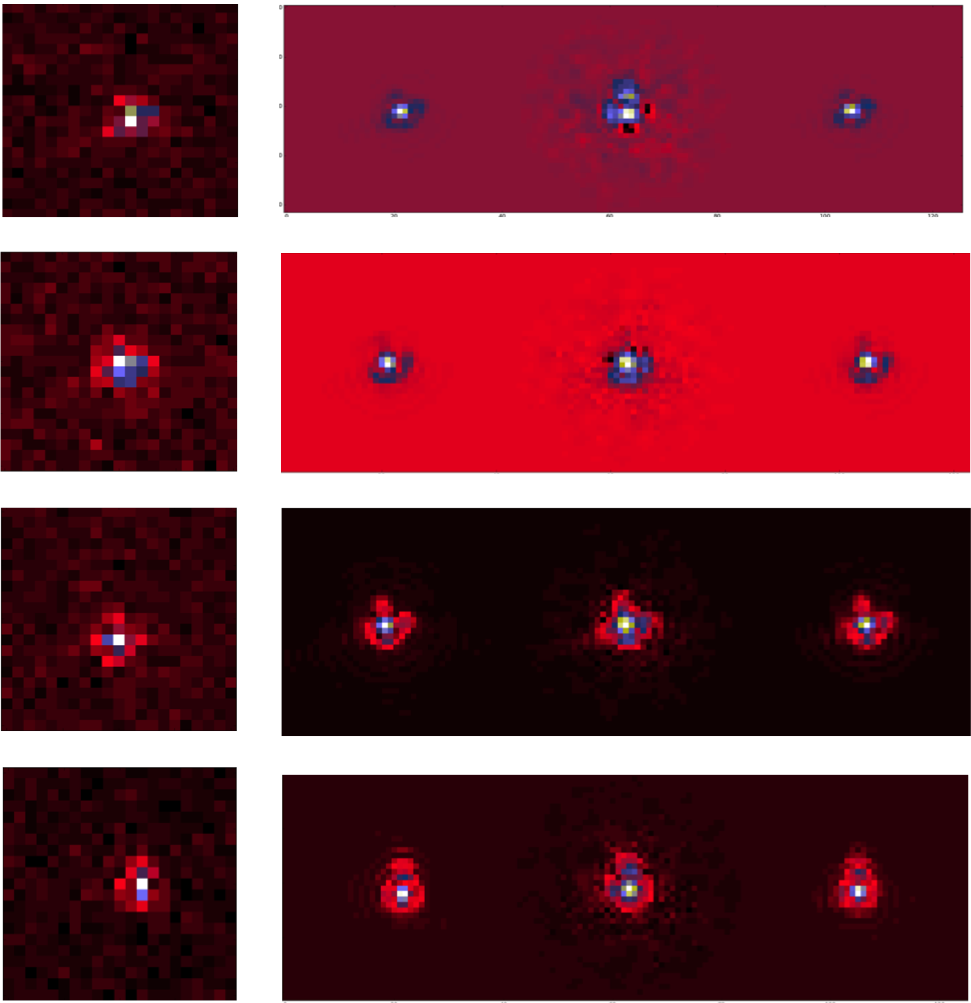## RCA

 Authors: F. Ngolè-Mboula Language: Python Download: GitHub Description: RCA: sparsity-based dimension reduction and super-resolution algorithm. Notes: Packaged version available here: rca.tar.gz

• RCA: sparsity-based dimension reduction and super-resolution algorithm.

Python source code available here: rca.tar.gz

## The method

RCA (Resolved Components Analysis, ) aims at characterizing globally a space-variant PSFs field. Given a set of aliased and noisy images of unresolved objects (stars images from a telescope for instance), RCA estimates well-resolved and noise-free PSFs at the observations positions, in particular, exploiting the spatial correlation of the PSFs across the imaging system field of view (FOV). Let consider a set of p images of unresolved objects $$\mathbf{y}_1,\dots,\mathbf{y}_p$$ in a given instrument FOV and $$\mathbf{x}_1,\dots,\mathbf{x}_p$$ the corresponding ideal PSFs; these images are treated as column vectors and we note $$\mathbf{Y} = [\mathbf{y}_1,\dots,\mathbf{y}_p]$$ and $$\mathbf{X} = [\mathbf{x}_1,\dots,\mathbf{x}_p]$$. RCA minimizes $$\frac{1}{2}{\|\mathbf{Y}-\mathcal{F}(\mathbf{X})\|_F^2}$$, where $$\mathcal{F}$$ accounts for the observed stars downsampling; this is done subject to several constraints over $$\mathbf{X}$$:

• Positivity constraint: each PSF $$\mathbf{x}_j$$ should be positive;
• Low rank constraint: each estimated PSF is forced to be a linear combination of a few number esimated of "eigen" PSFs; $$\mathbf{x}_j = \sum_{i=1}^r a_{ij} \mathbf{s}_i$$, with $$r \ll p$$ or equivalently, $$\mathbf{X} = \mathbf{S}\mathbf{A}$$, $$\mathbf{S}$$ and $$\mathbf{A}$$ being low rank matrices;
• Piece-wise smoothness constraint: we can assume that the vectors $$\mathbf{x}_j$$ are structured; promoting the sparsity of the "eigen" PSFs $$\mathbf{s}_j$$ in an appropriate dictionary $$\boldsymbol{\Phi}$$ allows to capture the spatial correlations within the PSFs themselves;
• Proximity constraints: the more two PSFs are close in the FOV, the more they should be similar; the p values relative to the $$i^{th}$$ line of $$\mathbf{A}$$ correspond to the contribution of the $$i^{th}$$ "eigen" PSF across the field of view; therefore the PSFs field regularity is enforced by constraining $$\mathbf{A}$$'s lines; specifically, each line is calculated as a sparse linear combination of spatial frequencies atoms: $$\mathbf{A} = \boldsymbol{\alpha}\mathbf{U}^T$$ (see Fig.1).Figure 1: Data matrix factorization. $$\mathbf{U}$$ columns correspond to different spatial frequencies of the PSFs field.

Hence RCA solves the following problem:
$\underset{\boldsymbol{\alpha},\mathbf{S}}\min \frac{1}{2}{\|\mathbf{Y}-\mathcal{F}(\mathbf{S}\mathbf{A})\|_F^2} + \sum_{i=1}^r \|\mathbf{w}_i\odot\boldsymbol{\Phi}\odot\mathbf{s}_i\|_1\; \text{s.t.}\; \|\boldsymbol{\alpha}[l,:]\|_0 \leq \eta_l, \; l=1....r \;\text{and } \mathbf{S}\boldsymbol{\alpha}\mathbf{U}^T \geq 0.$

The operator $$\mathcal{F}$$ is set according to the resolution enhancement factor specified. Besides, this operator also accounts for the observed images subpixel offsets which are automatically estimated. $$\boldsymbol{\Phi}$$ is a user-chosen redundant dictionary. The frequency dictionary $$\mathbf{U}$$ is automatically built based on the observations $$\mathbf{y}_i$$ locations in the imaging instrument FOV. The sparsity parameters $$(\mathbf{w}_i)_i$$ and $$(\eta_l)_l$$ as well are automatically selected. The number of "eigen" PSFs, r, has to be specified. It might be automatically reduced depending of the signal-to-noise ratio, the observations resolution and the sample size. A principal components analysis should provide the user a good first guess for r.

## Numerical examples

RCA was tested on set on 500 Euclid telescope simulated PSFs. The simulated observables were downsampled to Euclid telescope resolution and corrupted with a white gaussian noise. Four examples of recovered PSFs are given in Fig.2. In this example, 10 "eigen" PSFs were required. We compare the result to the PSFs obtained from the software PSFEx, using the same observations.Figure 2: Euclid PSFs restoration. From the left to the right: observed PSFs, original PSFs, PSFEx restored PSFs, RCA restored PSFs.

As it can be seen from these reconstructions, RCA handles the undersampling and the PSFs spatial variability, with both noise-robustness and a high visual quality.

## Article

•  F. M. Ngolè Mboula, J.-L. Starck, K. Okomura, J. Amiaux, P. Hudelot. Constraint matrix factorization for space variant PSFs field restoration, Inverse Problems. Available here.

## SPRITE

 Authors: F. Ngolè-Mboula Language: C++ Download: sprite_v1.tgz Description: SPRITE: sparsity-based super-resolution algorithm Notes:

SPRITE: sparsity-based super-resolution algorithm

## The method

SPRITE (Sparse Recovery of InstrumenTal rEsponse, 1) aims at computing a well-resolved compact source image from several undersampled and noisy observations. SPRITE solves the succession of problems of the form
$\underset{\Delta}\min \frac{1}{2}\sum_{k=1}^n{\|\mathbf{y}_k-f_k\mathbf{D}\mathbf{H}_k(\Delta+\mathbf{x}^{(0)})\|_2^2}/{\sigma_k^2} +\kappa\|\mathbf{w}^{(l)}\odot\Lambda\odot\Phi\Delta\|_1 ; s.t. \Delta \ge -\mathbf{x}^{(0)}, with l=1..N$.
The vectors $$\mathbf{y}_k$$ are the low resolution (LR) observations. The scalars $$f_k$$ account for possible luminosity differences between the LR images and $$\sigma_k^2$$ is the noise variance in the LR image $$k^{th}$$. The matrices $$\mathbf{H}_k$$ account for the shifts between the observations and the matrix D is the downsampling operator. The vector $$\mathbf{x}^{(0)}$$ is a rough estimate of the well-resolved image. The final image is given by $$\mathbf{x}=\mathbf{x}^{(0)}+\Delta_N$$, where $$\Delta_N$$ is the minimizer of the $$N^{th}$$ problem solved.

$$\Phi$$ is a user-chosen redundant dictionary. The method relies on the prior that a suitable solution $$\Delta$$ should have a sparse decomposition into the dictionary $$\Phi$$. Finally the inequality constraint (which is element-wise) insures that the final well-resolved image has positive pixels values.

It's important to note that the only parameters to be provided by the user are $Phi$ and $kappa$. The other parameters are automatically calculated.

The source code can be downloaded here: SPRITE package. The data and IDL codes used to perform the benchmark tests presented in 1 are available here: super-resolution benchmark.

Please cite the reference below if you use these codes in a publication. This is a preliminary version of the SPRITE package, which may be imperfect. Please feel free to contact us if you have any problem.

## Article

•  F. M. Ngolè Mboula, J.-L. Starck, S. Ronayette, K. Okomura, J. Amiaux. Super-resolution method using sparse regularization for point spread function recovery, Astronomy and Astrophysics, 2014. Available here.

## SF_Deconvolve

 Authors: S. Farrens Language: Python Download: GitHub Description: A Python code designed for PSF deconvolution using a low-rank approximation and sparsity. The code can handle a fixed PSF for the entire field or a stack of PSFs for each galaxy position. Notes: This code was used to produce the results presented in the paper Space variant deconvolution of galaxy survey images. Sample Euclid-like PSF data can be downloaded from here [63Mb] Sample galaxy images can be downloaded from here [5.5Mb]

## Introduction

The following sections provide some details for how to run sf_deconvolve.

The directory lib contains all of the primary functions and classes used for optimisation and analysis. functions contains some additional generic functions and tools.

## Dependencies

In order to run the code in this repository the following packages must be installed:

• Python 2.7 [Tested with v 2.7.11]
• Numpy [Tested with v 1.11.3]
• Scipy [Tested with v 0.18.1]
• Astropy [Tested with v 1.1.2]
• Matplotlib [Tested with v 1.5.3]
• Termcolor [Tested with v 1.1.0]

The current implementation of wavelet transformations additionally requires the mr_transform.cc C++ script from the Sparse2D library in the iSap package [Tested with v 3.1]. These C++ scripts will be need to be compiled in order to run (see iSap Documentation for details).

The low-rank approximation method can be run purely in Python.

## Execution

The primary code is sf_deconvolve.py which is designed to take an observed (i.e. with PSF effects and noise) stack of galaxy images and a known PSF, and attempt to reconstruct the original images. The input format are Numpy binary files (.npy) or FITS image files (.fits).

The code can be run as follows:

$sf_deconvolve.py -i INPUT_IMAGES.npy -p PSF.npy -o OUTPUT_NAME Where INPUT_IMAGES.npy denotes the Numpy binary file containing the stack of observed galaxy images, PSF.npy denotes the PSF corresponding to each galaxy image and OUTPUT_NAME specifies the output path and file name. Alternatively the code arguments can be stored in a configuration file (with any name) and the code can be run by providing the file name preceded by a @. $ sf_deconvolve.py @config.ini

### Example

The following example can be run on the sample data provided in the example directory.

This example takes a sample of 100 galaxy images (with PSF effects and added noise) and the corresponding PSFs, and recovers the original images using low-rank approximation via Condat-Vu optimisation.

$sf_deconvolve.py -i example_image_stack.npy -p example_psf.npy -o example_output --mode lowr  The example can also be run using the configuration file provided. The result will be two Numpy binary files called example_output_primal.npy and example_output_dual.npy corresponding to the primal and dual variables in the splitting algorithm. The reconstructed images will be in the example_output_primal.npy file. ### Code Options Required Arguments -i INPUT, --input INPUT: Input data file name. File should be a Numpy binary containing a stack of noisy galaxy images with PSF effects (i.e. a 3D array). -p PSF, --psf PSF: PSF file name. File should be a Numpy binary containing either: (a) a single PSF (i.e. a 2D array for fixed format) or (b) a stack of PSFs corresponding to each of the galaxy images (i.e. a 3D array for obj_var format). Optional Arguments -h, --help: Show the help message and exit. -v, --version: Show the program's version number and exit. -q, --quiet: Suppress verbose for each iteration. -o, --output: Output file name. If not specified output files will placed in input file path. --output_format Output file format [npy or fits]. Initialisation -k, --current_res: Current deconvolution results file name (i.e. the file containing the primal results from a previous run). --noise_est: Initial estimate of the noise standard deviation in the observed galaxy images. If not specified this quantity is automatically calculated using the median absolute deviation of the input image(s). Optimisation -m, --mode {all,sparse,lowr,grad}: Option to specify the optimisation mode [all, sparse, lowr or grad]. all performs optimisation using both low-rank approximation and sparsity, sparse using only sparsity, lowr uses only low-rank and grad uses only gradient descent. (default: lowr) --opt_type {condat,fwbw,gfwbw}: Option to specify the optimisation method to be implemented [condat, fwbw or gfwbw]. condat implements the Condat-Vu proximal splitting method, fwbw implements Forward-Backward splitting with FISTA speed-up and gfwbw implements the generalised Forward-Backward splitting method. (default: condat) --n_iter: Number of iterations. (default: 150) --cost_window: Window to measure cost function (i.e. interval of iterations for which cost should be calculated). (default: 1) --convergence: Convergence tolerance. (default: 0.0001) --no_pos: Option to turn off positivity constraint. --no_grad: Option to turn off gradient calculation. Low-Rank Aproximation --lowr_thresh_factor: Low rank threshold factor. (default: 1) --lowr_type: Type of low-rank regularisation [standard or ngole]. (default: standard) --lowr_thresh_type: Low rank threshold type [soft or hard]. (default: hard) Sparsity --wavelet_type: Type of Wavelet to be used (see iSap Documentation). (default: 1) --wave_thresh_factor: Wavelet threshold factor. (default: [3.0, 3.0, 4.0]) --n_reweights: Number of reweightings. (default: 1) Condat Algorithm --relax: Relaxation parameter (rho_n in Condat-Vu method). (default: 0.8) --condat_sigma: Condat proximal dual parameter. (default: 0.5) --condat_tau: Condat proximal primal parameter. (default: 0.5) Testing -c, --clean_data: Clean data file name. -r, --random_seed: Random seed. Use this option if the input data is a randomly selected subset (with known seed) of the full sample of clean data. --kernel: Standard deviation of pixels for Gaussian kernel. This option will multiply the deconvolution results by a Gaussian kernel. --metric: Metric to average errors [median or mean]. (default: median) ## Troubleshooting If you get the following error: ERROR: svd() got an unexpected keyword argument 'lapack_driver'  Update your Numpy and Scipy installations $ pip install --upgrade numpy
\$ pip install --upgrade scipy